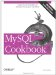# Converting Two-Digit Year Values to Four-Digit Form

10.30.1 Problem

You need to convert years in date values from two digits to four digits.

10.30.2 Solution

Let MySQL do this for you. If MySQL's conversion rules aren't appropriate, perform the operation yourself.

10.30.3 Discussion

Two-digit year values are a problem because the century is not explicit in the data values. If you know the range of years spanned by your input, you can add the century without ambiguity. Otherwise, you can only guess. For example, the date 2/10/69 probably would be interpreted by most people in the U.S. as as October 2, 1969. But if it represents Mahatma Gandhi's birth date, the year actually is 1869.

One way to convert years to four digits is to let MySQL do it. If you store a date containing a two-digit year, MySQL automatically converts it to four-digit form. MySQL uses a transition point of 1970; it interprets values from 00 to 69 as the years 2000 to 2069, and values from 70 to 99 as the years 1970 to 1999. These rules are appropriate for year values in the range from 1970 to 2069. If your values lie outside this range, you should add the proper century yourself before storing them into MySQL.

To use a different transition point, convert years to four-digit form yourself. A general purpose routine to convert two-digit years to four digits and to allow an arbitrary transition point can be written like this:

```sub yy_to_ccyy
{
my (\$year, \$transition_point) = @_;

\$transition_point = 70 unless defined (\$transition_point);
\$year += (\$year >= \$transition_point ? 1900 : 2000) if \$year < 100;
return (\$year);
}```

The function uses MySQL's transition point (70) by default. An optional second argument may be given to provide a different transition point. yy_to_ccyy( ) also makes sure the year actually needs converting (is less than 100) before modifying it. That way you can pass year values that do or don't include the century without checking first. Some sample invocations using the default transition point have the following results:

```\$val = yy_to_ccyy (60); # returns 2060
\$val = yy_to_ccyy (1960); # returns 1960 (no conversion done)```

But suppose you want to convert year values as follows, using a transition point of 50:

```00 .. 49 -> 2000 .. 2049
50 .. 99 -> 1950 .. 1999```

To do this, pass an explicit transition point argument to yy_to_ccyy( ):

```\$val = yy_to_ccyy (60, 50); # returns 1960
\$val = yy_to_ccyy (1960, 50); # returns 1960 (no conversion done)```

The yy_to_ccyy( ) function is one of those included in the Cookbook_Utils.pm library file.MySQL Cookbook
ISBN: 059652708X
EAN: 2147483647
Year: 2005
Pages: 412
Authors: Paul DuBois